# Basic plots

To start, we will use the iris dataset that is built into tidyverse/ggplot2. To view the dataset, use the `View()` command like so:

``View(iris)``

Once we have this, let’s setup a basic boxplot of some of the features of iris.

The iris dataset is built into tidyverse/ggplot2. The dataset is a pretty famous dataset by Edgar Anderson that gives the sepal length, width and petal length and width for three species of iris (n=50).

We are going to begin by plotting the sepal length for each species in a basic boxplot.

``````iris.box <- ggplot(iris, aes(x=Species,y=Sepal.Length)) +
geom_boxplot()

iris.box # We have to run a line with the name of the plot object to view the graph. ``````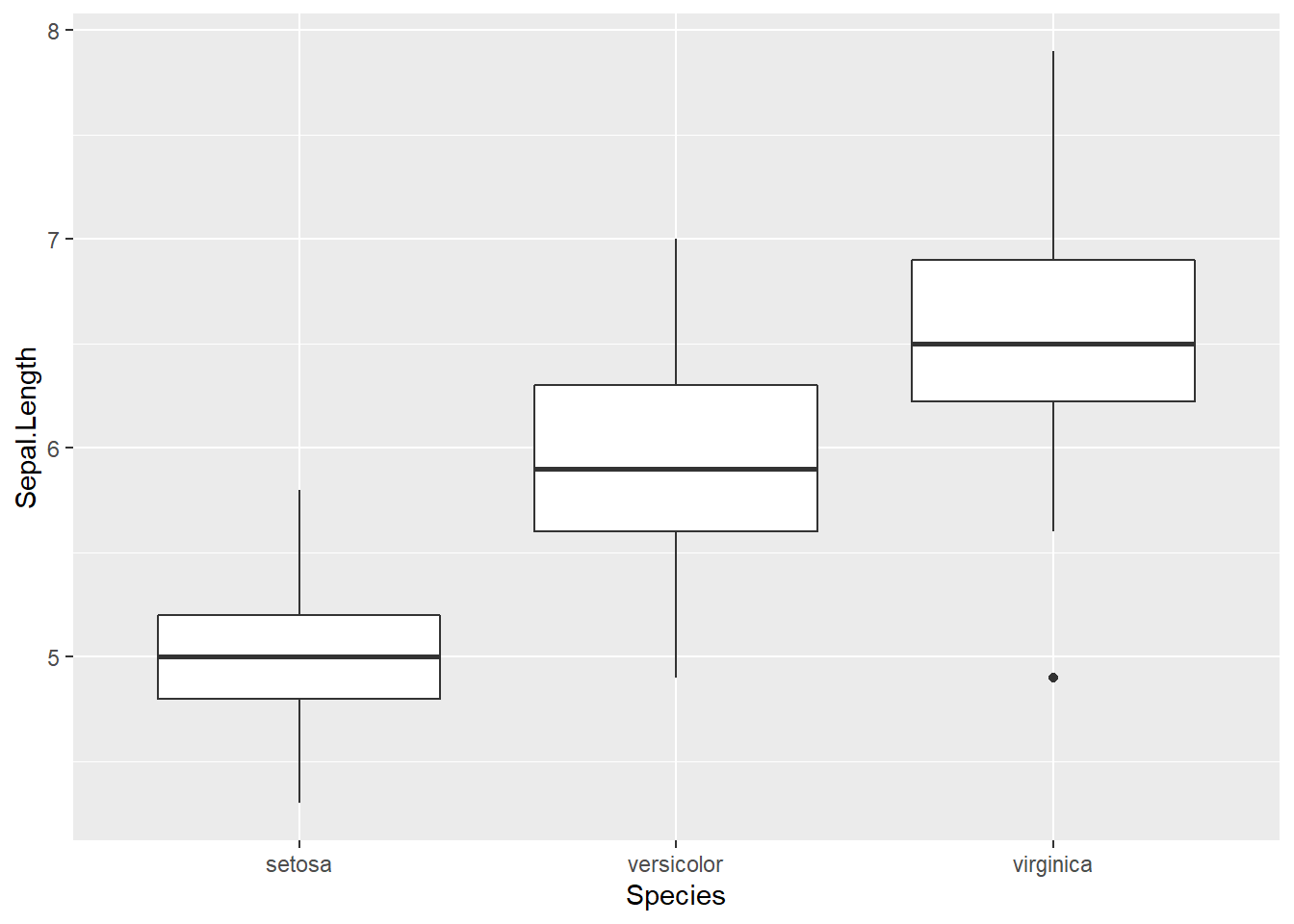So far, pretty straight forward.

You will notice I saved the ggplot() graph to an object called iris.box. Because I saved the plot to an object, I have to run the object name to view the plot. This is identical to using the command `print(iris.box)`.

Now let’s look at some others, such as a histogram.

``````iris.hist <- ggplot(iris, aes(x=Sepal.Length)) +
geom_histogram()

iris.hist``````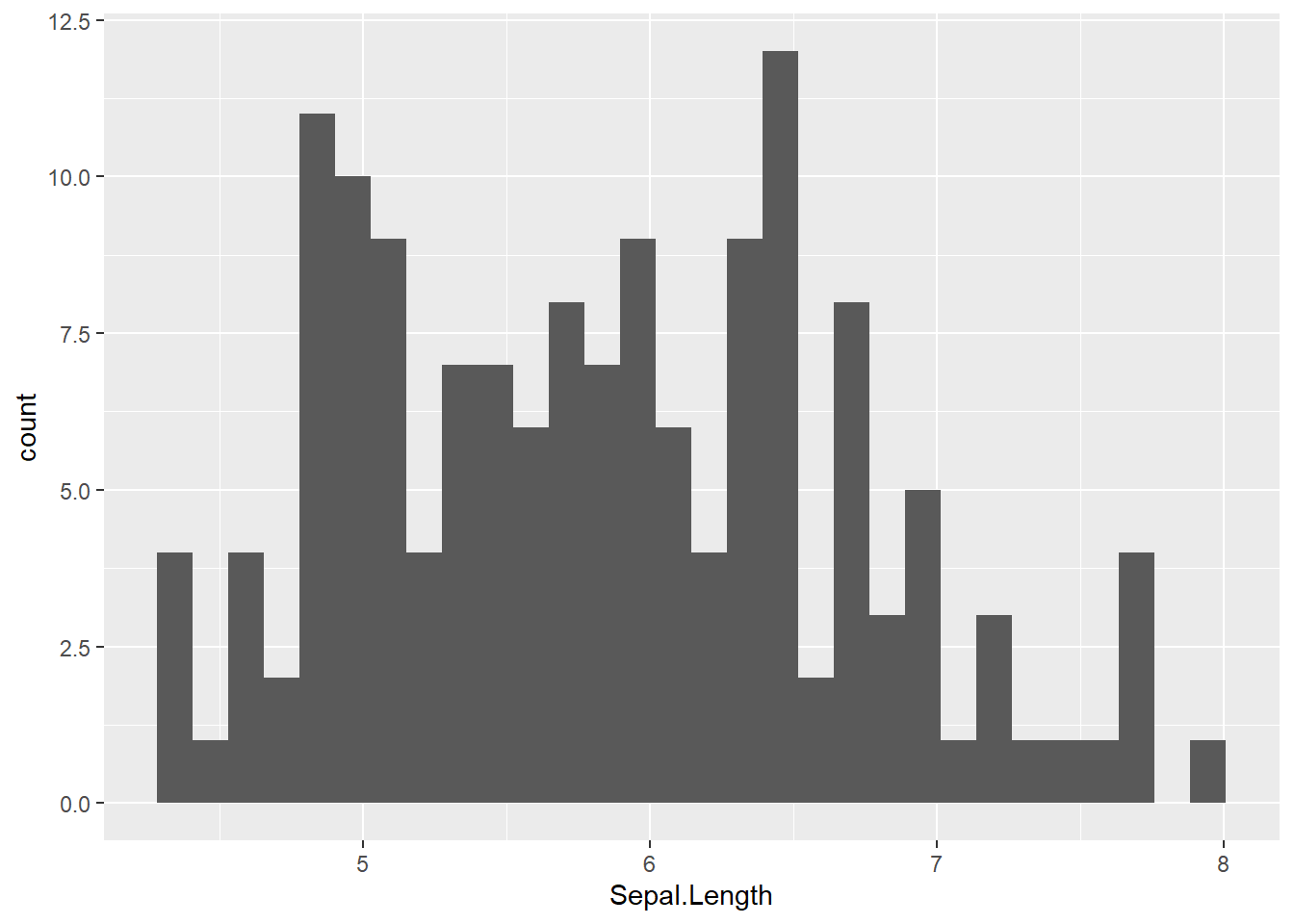That’s pretty ugly, but a simple addition of binwidth=“value” will fix that. Binwidth refers to the width of each bin, or bar, in the frequency histogram. A bin width of 0.5 means each bar of the histogram will be equal to 0.5 on the x axis (e.g. 4, 4.5, 5, 5.5 etc).

``````iris.hist <- ggplot(iris, aes(x=Sepal.Length)) +
geom_histogram(binwidth = 0.5)

iris.hist``````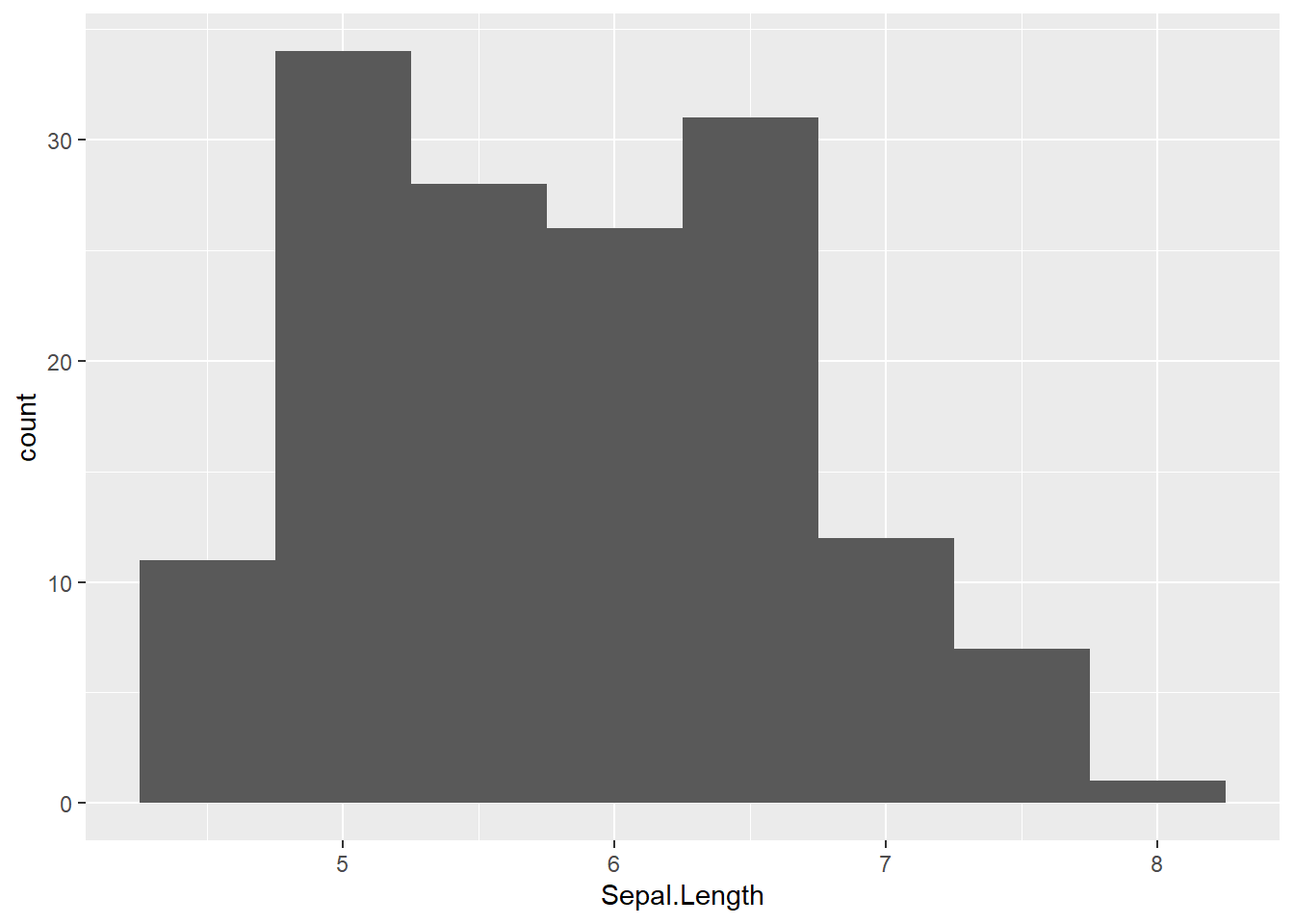Now let’s look at a scatterplot.

``````iris.scatter <- ggplot(iris, aes(x=Sepal.Length,y=Petal.Length)) +
geom_point()

iris.scatter``````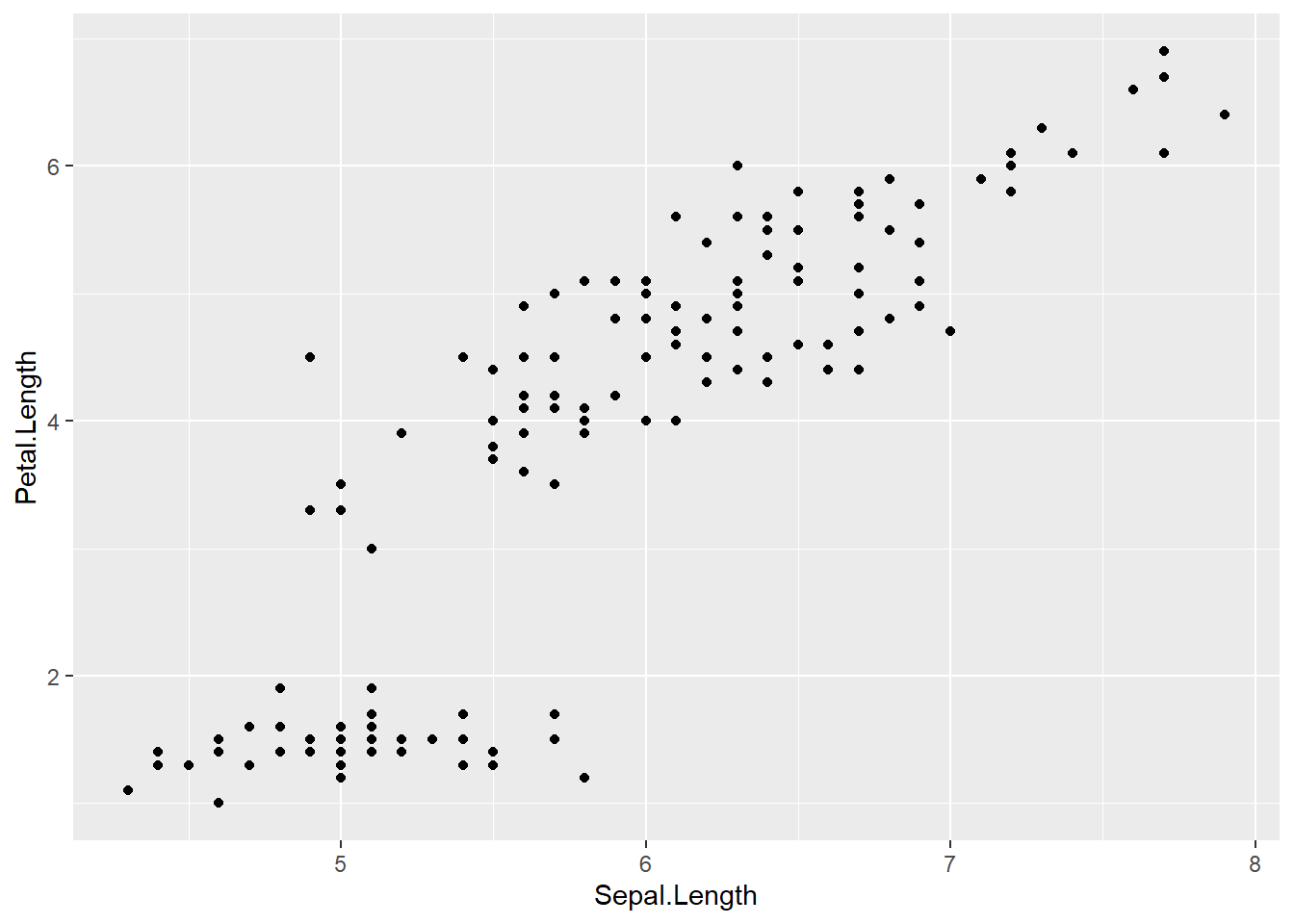The cool thing we can do with scatterplots is colour the points by a categorical feature such as Species. This is done by adding colour = “categorical variable name” in the aes brackets of the `ggplot()` command.

``````iris.scatter <- ggplot(iris, aes(x=Sepal.Length, y=Petal.Length, colour=Species)) +
geom_point()
iris.scatter``````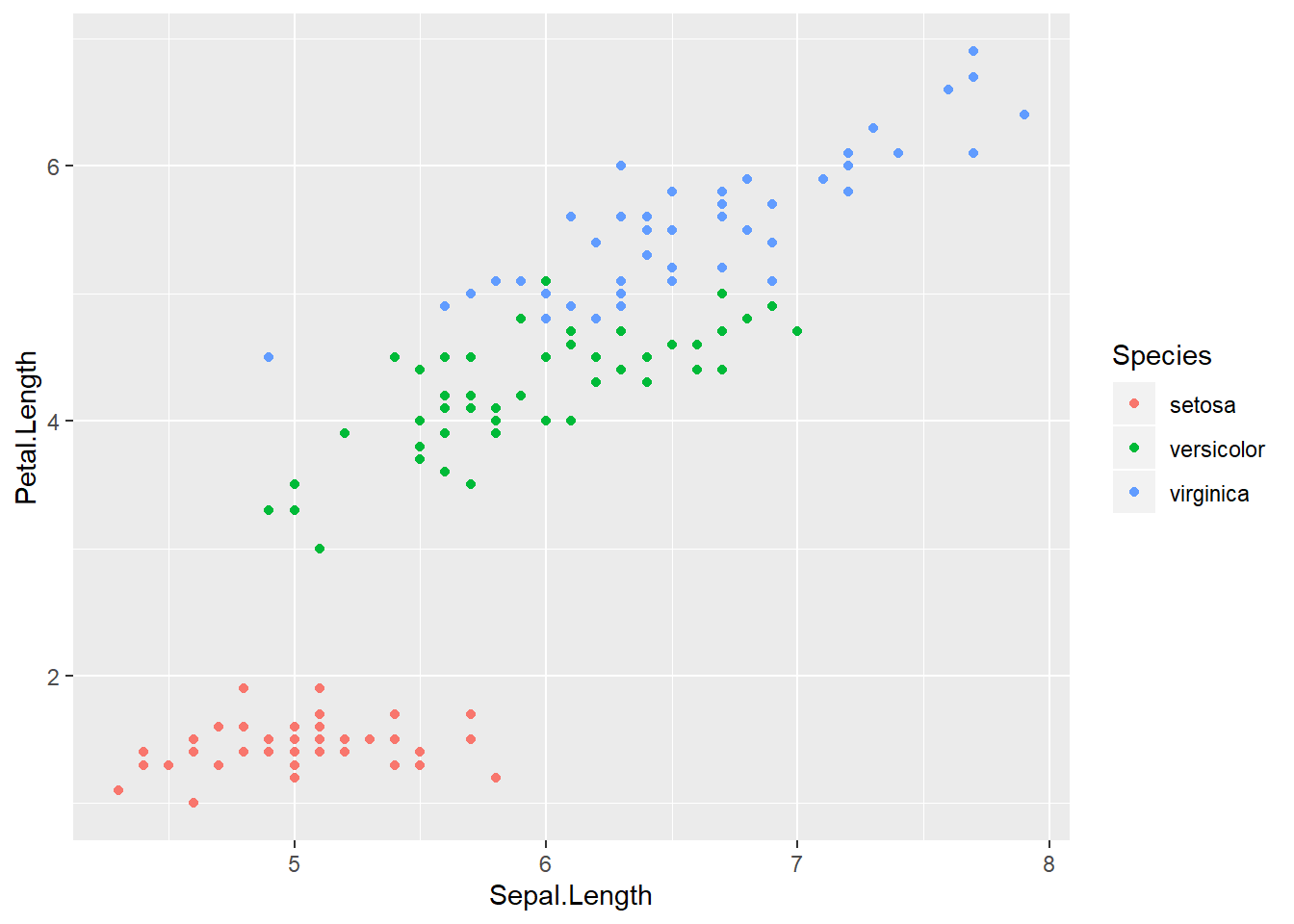Much better. And it even adds a legend for us.

Now we have this basic setup, we can start adding things to our graph. Due to the immense amount of customisations for our graphs, I will break these down in to sections as much as possible and explain as I go. We will work with the iris dataset for a while before moving to our analysed datasets.